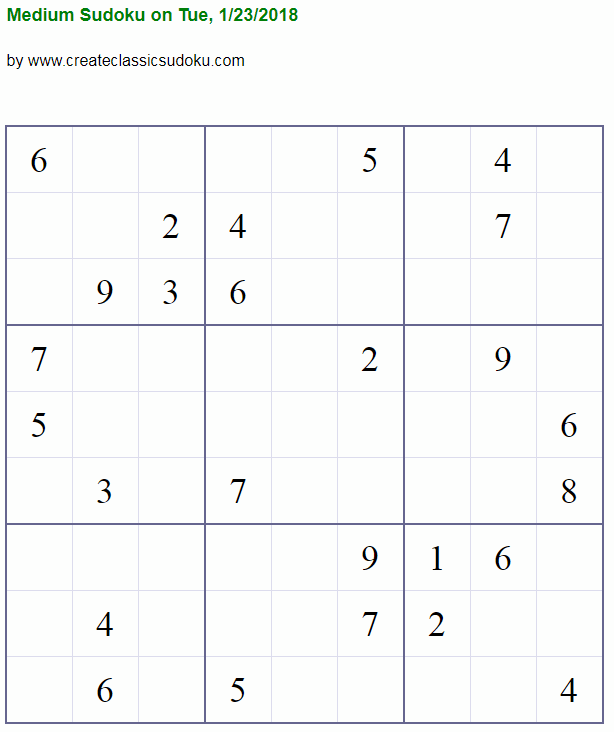Chat

# How Do I Solve a Sudoku Puzzle?

Sudoku is a number game in which missing numbers are to be filled into a 9 by 9 grid of squares which are subdivided into 3 by 3 boxes so that every row, every column, and every box contains the numbers 1 through 9.

Below is a Sudoku puzzle of medium difficulty level taking from www.createclassicsudoku.com.You are given a Sudoku puzzle, some of the squares are pre-filled with a number, and your task is to fill the remaining empty squares so that each row/column/box has nine different numbers 1 through 9.

How do I solve a Sudoku puzzle like this? Because this is a logical game, a lot of methods have been summarized to solve Sudoku puzzles, and more to come (you may be the one who will invent new solving methods). The most famous methods are OneChoice, Elimination, Interaction, Subset, Hidden Twins, X-Wing, XY-wing, XYZ-Wing, and guessing.

Here are examples of using OneChoice.

Here are examples of using Elimination.

Here are examples of using Subset.

Here are examples of using Hidden Pair/Hidden Twins.

Here are examples of using Interaction.

Here are examples of using X-Wing.

Here are examples of using XY-Wing.

Here are examples of using Guessing.

One book I like the most is Mensa Guide to Solving Sudoku by Peter Gordon. Almost all methods are summarized and plenty of exercises that will use certain methods are given.